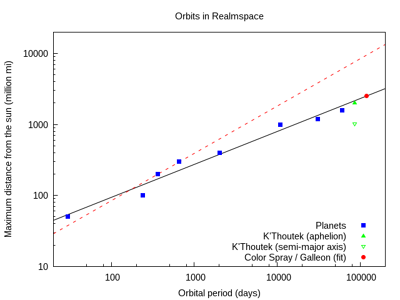Lately I haven't been able to contribute to the wiki as often as I'd like. Real life concerns tend to be… distracting sometimes.

But with a bit of free time available, I decided to write up a more detailed analysis of the astronomical properties of Realmspace. As a theoretical physicist specialized in gravitational physics and currently teaching Astronomy, I feel it is quite tempting to try to make some "early-astronomy" sense of the several celestial bodies and their movements in wildspace.

This is a follow-up on a recent discussion with Lhynard about the possible distance of the Color Spray Nebula from the sun. As far as I know, it is not stated in any official sources, but, as in the case of Neptune and Uranus in Earth's crystal sphere, maybe one can estimate its distance by looking at the other objects whose distances are known.

If one expects the planets in Realmspace to follow any kind of distance-period relation, the first guess as to which relation would be Kepler's third law, which states that the square of the orbital periodand the cube of the orbital semi-major axisare related to one another by the expression:However, the planets in Realmspace do not follow that relation. Which is not really surprising since gravity does not work in D&D the same way it does in real life. However, surprisingly, instead of just being peppered in random distances and periods, the planets do seem to follow a very discernible pattern.Realmspace orbital values. The red dashed line is Kepler's Third Law and the black solid line is the adjusted curve (see text).

More interestingly, the planets fall quite neatly into some sort of power-law behavior. That is, given a certain orbital period, one could predict the orbital radius by using an expression like this:Note also that one may write Kepler's Third Law in the same form, in which case the constantsandassume the values 1 (if you calibrate your distances in AU and periods in Earth years) and  23, respectively. So, in our case we need to find the exponentand the linear parameterthat best fit our data set.

Since this is a two-parameter curve, we may find the values ofandby performing linear regression on the data. What we find isAs can be seen in the figure above, the quality of this fit is pretty satisfactory (). By plugging these values in the equation above, we find that, if the Color Spray and Galleon nebulae follow the same pattern, they should lie at a distance of 2.5 billion miles (4 billion km) from the sun.

At this point, we could also check if this analysis holds for other objects orbiting the sun. Clearly, the Skull of the Void does not apply, since it is a fixed object, and Caer Windlauer's exact distances and orbital period are unknown. It turns out that comet K'Thoutek has known distance and period, so we could check if it falls neatly in line.

Again, we find another discrepancy with respect to Kepler's Third Law: it takes into account the semi-major axis of the orbit, which is roughly half of the comet's aphelion distance. Plugging that value into our equation gives the wrong answer, but plugging the actual aphelion distance reproduces "observational" data quite well.

Finally, it is worth mentioning how puzzling it is that celestial bodies in Realmspace follow a power law at all, and even more so that it is with a different exponent. Instead of the physical 0.67, we get 0.46. I wonder if the Realms' sages ever figured this out and named this law.